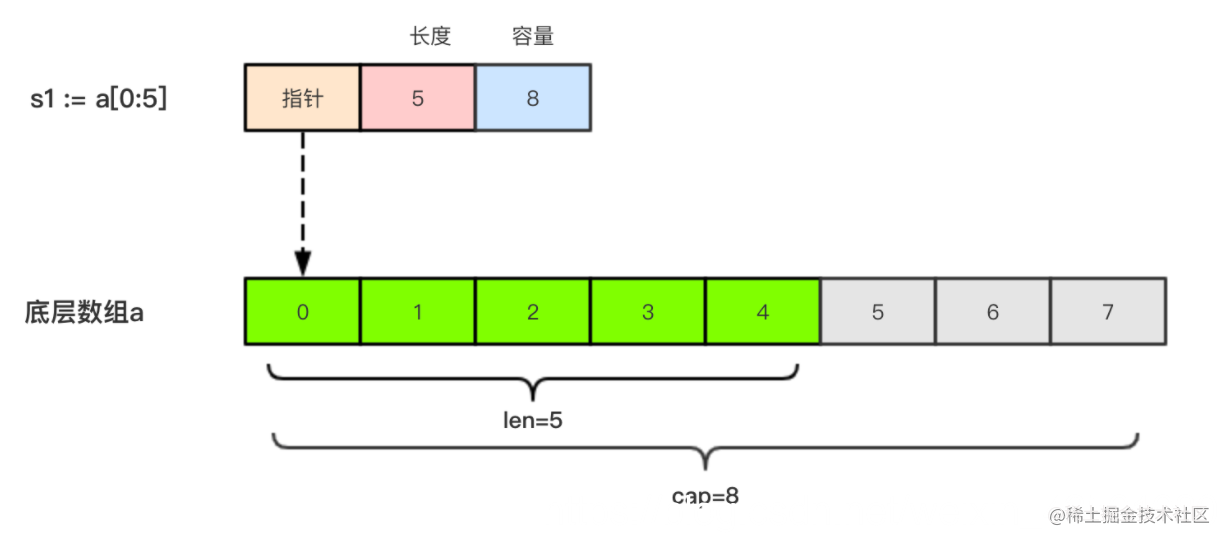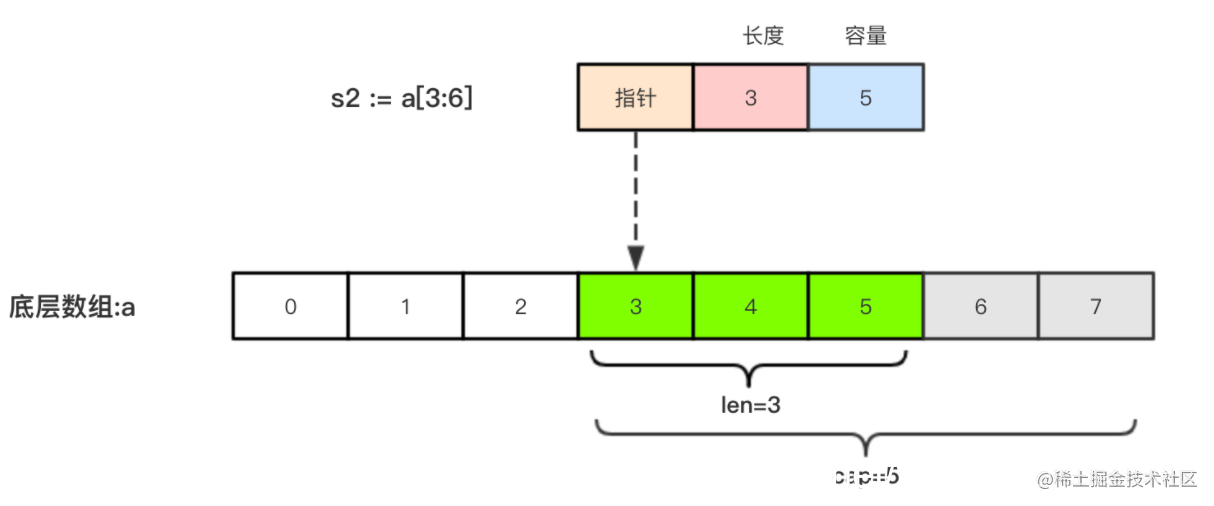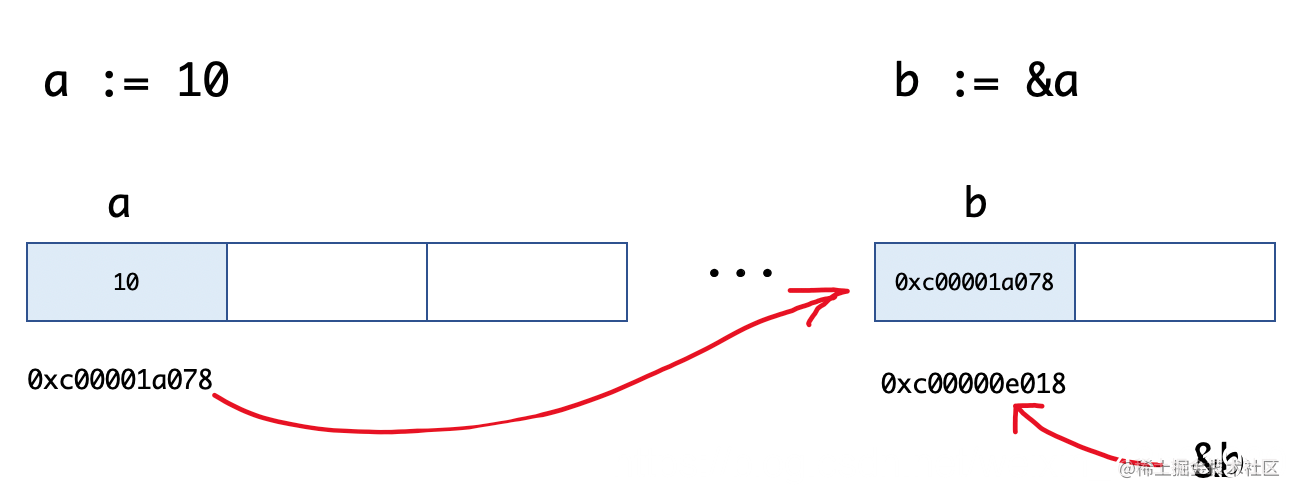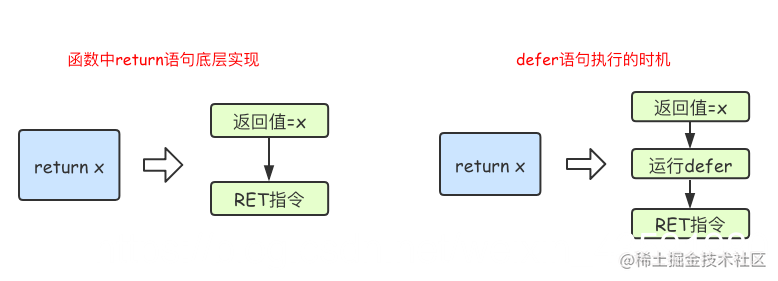# Go语言基本语法 (中)

Golang 中文文档地址：studygolang.com/pkgdoc
Go语言基本语法 (上)

### 1. 运算符

Go 语言内置的运算符有：

1. 算术运算符
2. 关系运算符
3. 逻辑运算符
4. 位运算符
5. 赋值运算符

+相加
-相减
*相乘
/相除
%求余

==检查两个值是否相等，如果相等返回 True 否则返回 False。
!=检查两个值是否不相等，如果不相等返回 True 否则返回 False。
>检查左边值是否大于右边值，如果是返回 True 否则返回 False。
>=检查左边值是否大于等于右边值，如果是返回 True 否则返回 False。
<检查左边值是否小于右边值，如果是返回 True 否则返回 False。
<=检查左边值是否小于等于右边值，如果是返回 True 否则返回 False。

&&逻辑 AND 运算符。 如果两边的操作数都是 True，则为 True，否则为 False。
||逻辑 OR 运算符。 如果两边的操作数有一个 True，则为 True，否则为 False。
!逻辑 NOT 运算符。 如果条件为 True，则为 False，否则为 True。

&参与运算的两数各对应的二进位相与。 （两位均为1才为1）
|参与运算的两数各对应的二进位相或。 （两位有一个为1就为1）
^参与运算的两数各对应的二进位相异或，当两对应的二进位相异时，结果为1。 （两位不一样则为1）
<<左移n位就是乘以2的n次方。 “a<<b”是把a的各二进位全部左移b位，高位丢弃，低位补0。
>>右移n位就是除以2的n次方。 “a>>b”是把a的各二进位全部右移b位。

=简单的赋值运算符，将一个表达式的值赋给一个左值
+=相加后再赋值
-=相减后再赋值
*=相乘后再赋值
/=相除后再赋值
%=求余后再赋值
<<=左移后赋值
>>=右移后赋值
&=按位与后赋值
|=按位或后赋值
^=按位异或后赋值

### 2. 数组

``````// 定义一个长度为3元素类型为int的数组a
var a int

##### 2.1 数组定义：
``````var 数组变量名 [元素数量]T

``````var a int
var b int
a = b //不可以这样做，因为此时a和b是不同的类型

#### 2.2 数组的初始化：

``````func main() {
var testArray int                        //数组会初始化为int类型的零值
var numArray = int{1, 2}                 //使用指定的初始值完成初始化
var cityArray = string{"北京", "上海", "深圳"} //使用指定的初始值完成初始化
fmt.Println(testArray)                      //[0 0 0]
fmt.Println(numArray)                       //[1 2 0]
fmt.Println(cityArray)                      //[北京 上海 深圳]
}

``````func main() {
var testArray int
var numArray = [...]int{1, 2}
var cityArray = [...]string{"北京", "上海", "深圳"}
fmt.Println(testArray)                          //[0 0 0]
fmt.Println(numArray)                           //[1 2]
fmt.Printf("type of numArray:%T\n", numArray)   //type of numArray:int
fmt.Println(cityArray)                          //[北京 上海 深圳]
fmt.Printf("type of cityArray:%T\n", cityArray) //type of cityArray:string
}

``````func main() {
a := [...]int{1: 1, 3: 5}
fmt.Println(a)                  // [0 1 0 5]
fmt.Printf("type of a:%T\n", a) //type of a:int
}

#### 2.3 数组的遍历：

``````func main() {
var a = [...]string{"北京", "上海", "深圳"}
// 方法1：for循环遍历
for i := 0; i < len(a); i++ {
fmt.Println(a[i])
}

// 方法2：for range遍历
for index, value := range a {
fmt.Println(index, value)
}
}

### 3. 多维数组

Go语言是支持多维数组的，我们这里以二维数组为例（数组中又嵌套数组）。

#### 3.1 二维数组的定义：

``````func main() {
a := string{
{"北京", "上海"},
{"广州", "深圳"},
{"成都", "重庆"},
}
fmt.Println(a) //[[北京 上海] [广州 深圳] [成都 重庆]]
fmt.Println(a) //支持索引取值:重庆
}

#### 3.2 二维数组的遍历：

``````func main() {
a := string{
{"北京", "上海"},
{"广州", "深圳"},
{"成都", "重庆"},
}
for _, v1 := range a {
for _, v2 := range v1 {
fmt.Printf("%s\t", v2)
}
fmt.Println()
}
}

``````北京	上海

``````//支持的写法
a := [...]string{
{"北京", "上海"},
{"广州", "深圳"},
{"成都", "重庆"},
}
//不支持多维数组的内层使用...
b := [...]string{
{"北京", "上海"},
{"广州", "深圳"},
{"成都", "重庆"},
}

#### 3.4 数组是值类型

``````func modifyArray(x int) {
x = 100
}

func modifyArray2(x int) {
x = 100
}
func main() {
a := int{10, 20, 30}
modifyArray(a) //在modify中修改的是a的副本x
fmt.Println(a) //[10 20 30]
b := int{
{1, 1},
{1, 1},
{1, 1},
}
modifyArray2(b) //在modify中修改的是b的副本x
fmt.Println(b)  //[[1 1] [1 1] [1 1]]
}

1. 数组支持 “==“、”!=” 操作符，因为内存总是被初始化过的。
2. `[n]*T`表示指针数组，`*[n]T`表示数组指针 。

``````package main

import "fmt"

func main() {
// 数组的定义
var a1 bool
fmt.Println(a1) // [false false false]
var a2 bool
fmt.Printf("a1:%T  a2:%T\n", a1, a2)

// 数组的初始化
a1 = bool{true,true,false}// 方式 1
fmt.Println(a1)
a3 := [...]int{1,2,3,4,5,6,7,8,9}// 方式 2
fmt.Println(a3)
a4 := int{1,2}// 方式 3
fmt.Println(a4)// [1 2 0 0 0]
a5 := int{0:1,4:2}// 方式 4
fmt.Println(a5)// [1 0 0 0 2]

// 数组的遍历
citys := [...]string{"北京","上海","深圳"}
// 1.根据索引遍历
for i:=0;i<len(citys);i++ {
fmt.Println(citys[i])
}
// 2.for range遍历
for index,value := range citys{
fmt.Println(index,value)
}

// 多维数组
var arr int
arr = int{
int{1,2},
int{3,4},
int{5,6},
}
fmt.Println(arr)

// 多维数组的遍历
for _,value := range arr{
for _,v := range value{
fmt.Println(v)
}
}

// 数组是值类型
b1 := int{1,2,3}
b2 := b1
b2 = 100
fmt.Println(b1,b2)// [1 2 3] [100 2 3]
}

### 4. 切片

#### 4.1 引子

``````func arraySum(x int) int{
sum := 0
for _, v := range x{
sum = sum + v
}
return sum
}

``````a := int{1, 2, 3}

#### 4.2 切片声明语法

``````var name []T

• `name`：表示变量名
• `T`：表示切片中的元素类型

``````func main() {
// 声明切片类型
var a []string              //声明一个字符串切片
var b = []int{}             //声明一个整型切片并初始化
var c = []bool{false, true} //声明一个布尔切片并初始化
var d = []bool{false, true} //声明一个布尔切片并初始化
fmt.Println(a)              //[]
fmt.Println(b)              //[]
fmt.Println(c)              //[false true]
fmt.Println(a == nil)       //true
fmt.Println(b == nil)       //false
fmt.Println(c == nil)       //false
// fmt.Println(c == d)   //切片是引用类型，不支持直接比较，只能和nil比较
}

#### 4.4 切片表达式

``````func main() {
a := int{1, 2, 3, 4, 5}
s := a[1:3]  // s := a[low:high]
fmt.Printf("s:%v len(s):%v cap(s):%v\n", s, len(s), cap(s))
}

``````s:[2 3] len(s):2 cap(s):4

``````a[2:]  // 等同于 a[2:len(a)]
a[:3]  // 等同于 a[0:3]
a[:]   // 等同于 a[0:len(a)]

``````func main() {
a := int{1, 2, 3, 4, 5}
s := a[1:3]  // s := a[low:high]
fmt.Printf("s:%v len(s):%v cap(s):%v\n", s, len(s), cap(s))
s2 := s[3:4]  // 索引的上限是cap(s)而不是len(s)
fmt.Printf("s2:%v len(s2):%v cap(s2):%v\n", s2, len(s2), cap(s2))
}

``````s:[2 3] len(s):2 cap(s):4
s2: len(s2):1 cap(s2):1

``````a[low : high : max]

``````func main() {
a := int{1, 2, 3, 4, 5}
t := a[1:3:5]
fmt.Printf("t:%v len(t):%v cap(t):%v\n", t, len(t), cap(t))
}

``````t:[2 3] len(t):2 cap(t):4

#### 4.5 使用make()函数构造切片

``````make([]T, size, cap)

• `T`: 切片的元素类型
• `size`: 切片中元素的数量
• `cap`: 切片的容量

``````func main() {
a := make([]int, 2, 10)
fmt.Println(a)      //[0 0]
fmt.Println(len(a)) //2
fmt.Println(cap(a)) //10
}

#### 4.6 切片的本质#### 4.8 切片不能直接比较

``````var s1 []int         //len(s1)=0;cap(s1)=0;s1==nil
s2 := []int{}        //len(s2)=0;cap(s2)=0;s2!=nil
s3 := make([]int, 0) //len(s3)=0;cap(s3)=0;s3!=nil

#### 4.9 切片的赋值拷贝

``````func main() {
s1 := make([]int, 3) //[0 0 0]
s2 := s1             //将s1直接赋值给s2，s1和s2共用一个底层数组
s2 = 100
fmt.Println(s1) //[100 0 0]
fmt.Println(s2) //[100 0 0]
}

#### 4.10 切片遍历

``````func main() {
s := []int{1, 3, 5}

for i := 0; i < len(s); i++ {
fmt.Println(i, s[i])
}

for index, value := range s {
fmt.Println(index, value)
}
}

#### 4.11 append()方法为切片添加元素

Go语言的内建函数`append()`可以为切片动态添加元素。 可以一次添加一个元素，可以添加多个元素，也可以添加另一个切片中的元素（后面加…）。

``````func main(){
var s []int
s = append(s, 1)        // 
s = append(s, 2, 3, 4)  // [1 2 3 4]
s2 := []int{5, 6, 7}
s = append(s, s2...)    // [1 2 3 4 5 6 7]
}

**注意：**通过var声明的零值切片可以在`append()`函数直接使用，无需初始化。

``````var s []int
s = append(s, 1, 2, 3)

``````s := []int{}  // 没有必要初始化
s = append(s, 1, 2, 3)

var s = make([]int)  // 没有必要初始化
s = append(s, 1, 2, 3)

``````func main() {
//append()添加元素和切片扩容
var numSlice []int
for i := 0; i < 10; i++ {
numSlice = append(numSlice, i)
fmt.Printf("%v  len:%d  cap:%d  ptr:%p\n", numSlice, len(numSlice), cap(numSlice), numSlice)
}
}

``````  len:1  cap:1  ptr:0xc0000a8000
[0 1]  len:2  cap:2  ptr:0xc0000a8040
[0 1 2]  len:3  cap:4  ptr:0xc0000b2020
[0 1 2 3]  len:4  cap:4  ptr:0xc0000b2020
[0 1 2 3 4]  len:5  cap:8  ptr:0xc0000b6000
[0 1 2 3 4 5]  len:6  cap:8  ptr:0xc0000b6000
[0 1 2 3 4 5 6]  len:7  cap:8  ptr:0xc0000b6000
[0 1 2 3 4 5 6 7]  len:8  cap:8  ptr:0xc0000b6000
[0 1 2 3 4 5 6 7 8]  len:9  cap:16  ptr:0xc0000b8000
[0 1 2 3 4 5 6 7 8 9]  len:10  cap:16  ptr:0xc0000b8000

1. `append()`函数将元素追加到切片的最后并返回该切片。
2. 切片numSlice的容量按照1，2，4，8，16这样的规则自动进行扩容，每次扩容后都是扩容前的2倍。

`append()`函数还支持一次性追加多个元素。 例如：

``````var citySlice []string
// 追加一个元素
citySlice = append(citySlice, "北京")
// 追加多个元素
citySlice = append(citySlice, "上海", "广州", "深圳")
// 追加切片
a := []string{"成都", "重庆"}
citySlice = append(citySlice, a...)
fmt.Println(citySlice) //[北京 上海 广州 深圳 成都 重庆]

#### 4.12 copy()函数复制切片

``````func main() {
a := []int{1, 2, 3, 4, 5}
b := a
fmt.Println(a) //[1 2 3 4 5]
fmt.Println(b) //[1 2 3 4 5]
b = 1000
fmt.Println(a) //[1000 2 3 4 5]
fmt.Println(b) //[1000 2 3 4 5]
}

Go语言内建的`copy()`函数可以迅速地将一个切片的数据复制到另外一个切片空间中，`copy()`函数的使用格式如下：

``````copy(destSlice, srcSlice []T)

• srcSlice: 数据来源切片
• destSlice: 目标切片

``````func main() {
// copy()复制切片
a := []int{1, 2, 3, 4, 5}
c := make([]int, 5, 5)
copy(c, a)     //使用copy()函数将切片a中的元素复制到切片c
fmt.Println(a) //[1 2 3 4 5]
fmt.Println(c) //[1 2 3 4 5]
c = 1000
fmt.Println(a) //[1 2 3 4 5]
fmt.Println(c) //[1000 2 3 4 5]
}

#### 4.13 从切片中删除元素

Go语言中并没有删除切片元素的专用方法，我们可以使用切片本身的特性来删除元素。 代码如下：

``````func main() {
// 从切片中删除元素
a := []int{30, 31, 32, 33, 34, 35, 36, 37}
// 要删除索引为2的元素
a = append(a[:2], a[3:]...)
fmt.Println(a) //[30 31 33 34 35 36 37]
}

### 5. 指针

#### 5.1 Go语言中的指针介绍

Go语言中的指针不能进行偏移和运算，因此Go语言中的指针操作非常简单，我们只需要记住两个符号：

• `&`：取地址
• `*`：根据地址取值

#### 5.2 指针地址和指针类型

``````ptr := &v    // v的类型为T

• v: 代表被取地址的变量，类型为`T`
• ptr: 用于接收地址的变量，ptr的类型就为`*T`，称做 T 的指针类型。*代表指针。

``````func main() {
a := 10
b := &a
fmt.Printf("a:%d ptr:%p\n", a, &a) // a:10 ptr:0xc00001a078
fmt.Printf("b:%p type:%T\n", b, b) // b:0xc00001a078 type:*int
fmt.Println(&b)                    // 0xc00000e018
}#### 5.3 指针取值

``````func main() {
//指针取值
a := 10
b := &a // 取变量a的地址，将指针保存到b中
fmt.Printf("type of b:%T\n", b)
c := *b // 指针取值（根据指针去内存取值）
fmt.Printf("type of c:%T\n", c)
fmt.Printf("value of c:%v\n", c)
}

``````type of b:*int
type of c:int
value of c:10

• 对变量进行取地址（`&`）操作，可以获得这个变量的指针变量。
• 指针变量的值是指针地址。
• 对指针变量进行取值（`*`）操作，可以获得指针变量指向的原变量的值。

``````func modify1(x int) {
x = 100
}

func modify2(x *int) {
*x = 100
}

func main() {
a := 10
modify1(a)
fmt.Println(a) // 10
modify2(&a)
fmt.Println(a) // 100
}

#### 5.4 new和make

``````func main() {
var a *int
*a = 100
fmt.Println(*a)

var b map[string]int
b["沙河娜扎"] = 100
fmt.Println(b)
}

##### new

new是一个内置的函数，它的函数签名如下：

``````func new(Type) *Type

• Type表示类型，new函数只接受一个参数，这个参数是一个类型
• *Type表示类型指针，new函数返回一个指向该类型内存地址的指针。

new函数不太常用，使用new函数得到的是一个类型的指针，并且该指针对应的值为该类型的零值。举个例子：

``````func main() {
a := new(int)
b := new(bool)
fmt.Printf("%T\n", a) // *int
fmt.Printf("%T\n", b) // *bool
fmt.Println(*a)       // 0
fmt.Println(*b)       // false
}

``````func main() {
var a *int
a = new(int)
*a = 10
fmt.Println(*a)
}

##### make

make也是用于内存分配的，区别于new，它只用于slice、map以及chan的内存创建，而且它返回的类型就是这三个类型本身，而不是他们的指针类型，因为这三种类型就是引用类型，所以就没有必要返回他们的指针了。make函数的函数签名如下：

``````func make(t Type, size ...IntegerType) Type

make 函数是无可替代的，我们在使用slice、map以及channel的时候，都需要使用make进行初始化，然后才可以对它们进行操作。这个我们在上一章中都有说明，关于channel我们会在后续的章节详细说明。

``````func main() {
var b map[string]int
b = make(map[string]int, 10)
b["沙河娜扎"] = 100
fmt.Println(b)
}

##### new与make的区别
1. 二者都是用来做内存分配的。
2. make只用于slice、map以及channel的初始化，返回的还是这三个引用类型本身
3. 而new用于类型的内存分配，并且内存对应的值为类型零值，返回的是指向类型的指针

### 6. Map

Go语言中提供的映射关系容器为`map`，其内部使用`散列表（hash）`实现。

#### 6.1 map 介绍

map是一种无序的基于`key-value`的数据结构，Go语言中的map是引用类型，必须初始化才能使用。

#### 6.2 map定义

Go语言中 `map`的定义语法如下：

``````map[KeyType]ValueType

• KeyType: 表示键的类型。
• ValueType: 表示键对应的值的类型。

map类型的变量默认初始值为nil，需要使用make()函数来分配内存。语法为：

``````make(map[KeyType]ValueType, [cap])

#### 6.3 map基本使用

map中的数据都是成对出现的，map的基本使用示例代码如下：

``````func main() {
scoreMap := make(map[string]int, 8)
scoreMap["张三"] = 90
scoreMap["小明"] = 100
fmt.Println(scoreMap)
fmt.Println(scoreMap["小明"])
fmt.Printf("type of a:%T\n", scoreMap)
}

``````map[小明:100 张三:90]
100
type of a:map[string]int

map也支持在声明的时候填充元素，例如：

``````func main() {
userInfo := map[string]string{
}
}

#### 6.4 判断某个键是否存在

Go语言中有个判断map中键是否存在的特殊写法，格式如下:

``````value, ok := map[key]

``````func main() {
scoreMap := make(map[string]int)
scoreMap["张三"] = 90
scoreMap["小明"] = 100
// 如果key存在ok为true,v为对应的值；不存在ok为false,v为值类型的零值
v, ok := scoreMap["张三"]
if ok {
fmt.Println(v)
} else {
fmt.Println("查无此人")
}
}

#### 6.5 map的遍历

Go语言中使用`for range`遍历map。

``````func main() {
scoreMap := make(map[string]int)
scoreMap["张三"] = 90
scoreMap["小明"] = 100
scoreMap["娜扎"] = 60
for k, v := range scoreMap {
fmt.Println(k, v)
}
}

``````func main() {
scoreMap := make(map[string]int)
scoreMap["张三"] = 90
scoreMap["小明"] = 100
scoreMap["娜扎"] = 60
for k := range scoreMap {
fmt.Println(k)
}
}

#### 6.6 delete()函数删除键值对

``````delete(map, key)

• map: 表示要删除键值对的map
• key: 表示要删除的键值对的键

``````func main(){
scoreMap := make(map[string]int)
scoreMap["张三"] = 90
scoreMap["小明"] = 100
scoreMap["娜扎"] = 60
delete(scoreMap, "小明")//将小明:100从map中删除
for k,v := range scoreMap{
fmt.Println(k, v)
}
}

#### 6.7 按照指定顺序遍历map

``````func main() {
rand.Seed(time.Now().UnixNano()) //初始化随机数种子

var scoreMap = make(map[string]int, 200)

for i := 0; i < 100; i++ {
key := fmt.Sprintf("stu%02d", i) //生成stu开头的字符串
value := rand.Intn(100)          //生成0~99的随机整数
scoreMap[key] = value
}
//取出map中的所有key存入切片keys
var keys = make([]string, 0, 200)
for key := range scoreMap {
keys = append(keys, key)
}
//对切片进行排序
sort.Strings(keys)
//按照排序后的key遍历map
for _, key := range keys {
fmt.Println(key, scoreMap[key])
}
}

#### 6.8 元素为map类型的切片

``````func main() {
var mapSlice = make([]map[string]string, 3)
for index, value := range mapSlice {
fmt.Printf("index:%d value:%v\n", index, value)
}
fmt.Println("after init")
// 对切片中的map元素进行初始化
mapSlice = make(map[string]string, 10)
mapSlice["name"] = "小王子"
for index, value := range mapSlice {
fmt.Printf("index:%d value:%v\n", index, value)
}
}

#### 6.9 值为切片类型的map

``````func main() {
var sliceMap = make(map[string][]string, 3)
fmt.Println(sliceMap)
fmt.Println("after init")
key := "中国"
value, ok := sliceMap[key]
if !ok {
value = make([]string, 0, 2)
}
value = append(value, "北京", "上海")
sliceMap[key] = value
fmt.Println(sliceMap)
}

### 7. 函数

Go语言中支持函数、匿名函数和闭包，并且函数在Go语言中属于“一等公民”。

#### 7.1 函数定义

Go语言中定义函数使用`func`关键字，具体格式如下：

``````func 函数名(参数)(返回值){
函数体
}

• 函数名：由字母、数字、下划线组成。但函数名的第一个字母不能是数字。在同一个包内，函数名也称不能重名（包的概念详见后文）。
• 参数：参数由参数变量和参数变量的类型组成，多个参数之间使用`,`分隔。
• 返回值：返回值由返回值变量和其变量类型组成，也可以只写返回值的类型，多个返回值必须用`()`包裹，并用`,`分隔。
• 函数体：实现指定功能的代码块。

``````func intSum(x int, y int) int {
return x + y
}

``````func sayHello() {
fmt.Println("Hello 沙河")
}

#### 7.2 函数的调用

``````func main() {
sayHello()
ret := intSum(10, 20)
fmt.Println(ret)
}

#### 7.3 参数

##### 7.3.1 类型简写

``````func intSum(x, y int) int {
return x + y
}

##### 7.3.2 可变参数

``````func intSum2(x ...int) int {
fmt.Println(x) //x是一个切片
sum := 0
for _, v := range x {
sum = sum + v
}
return sum
}

``````ret1 := intSum2()
ret2 := intSum2(10)
ret3 := intSum2(10, 20)
ret4 := intSum2(10, 20, 30)
fmt.Println(ret1, ret2, ret3, ret4) //0 10 30 60

``````func intSum3(x int, y ...int) int {
fmt.Println(x, y)
sum := x
for _, v := range y {
sum = sum + v
}
return sum
}

``````ret5 := intSum3(100)
ret6 := intSum3(100, 10)
ret7 := intSum3(100, 10, 20)
ret8 := intSum3(100, 10, 20, 30)
fmt.Println(ret5, ret6, ret7, ret8) //100 110 130 160

#### 7.4 返回值

Go语言中通过`return`关键字向外输出返回值。

##### 7.4.1 多返回值

Go语言中函数支持多返回值，函数如果有多个返回值时必须用`()`将所有返回值包裹起来。

``````func calc(x, y int) (int, int) {
sum := x + y
sub := x - y
return sum, sub
}

##### 7.4.2 返回值命名

``````func calc(x, y int) (sum, sub int) {
sum = x + y
sub = x - y
return
}

##### 7.4.3 返回值补充

``````func someFunc(x string) []int {
if x == "" {
return nil // 没必要返回[]int{}
}
...
}

#### 7.5 变量作用域

##### 7.5.1 全局变量

``````package main

import "fmt"

//定义全局变量num
var num int64 = 10

func testGlobalVar() {
fmt.Printf("num=%d\n", num) //函数中可以访问全局变量num
}
func main() {
testGlobalVar() //num=10
}

##### 7.5.2 局部变量

``````func testLocalVar() {
//定义一个函数局部变量x,仅在该函数内生效
var x int64 = 100
fmt.Printf("x=%d\n", x)
}

func main() {
testLocalVar()
fmt.Println(x) // 此时无法使用变量x
}

``````package main

import "fmt"

//定义全局变量num
var num int64 = 10

func testNum() {
num := 100
fmt.Printf("num=%d\n", num) // 函数中优先使用局部变量
}
func main() {
testNum() // num=100
}

``````func testLocalVar2(x, y int) {
fmt.Println(x, y) //函数的参数也是只在本函数中生效
if x > 0 {
z := 100 //变量z只在if语句块生效
fmt.Println(z)
}
//fmt.Println(z)//此处无法使用变量z
}

``````func testLocalVar3() {
for i := 0; i < 10; i++ {
fmt.Println(i) //变量i只在当前for语句块中生效
}
//fmt.Println(i) //此处无法使用变量i
}

#### 7.6 函数类型与变量

##### 7.6.1 定义函数类型

``````type calculation func(int, int) int

``````func add(x, y int) int {
return x + y
}

func sub(x, y int) int {
return x - y
}

``````var c calculation

##### 7.6.2 函数类型变量

``````func main() {
var c calculation               // 声明一个calculation类型的变量c
fmt.Printf("type of c:%T\n", c) // type of c:main.calculation

fmt.Printf("type of f:%T\n", f) // type of f:func(int, int) int
}

#### 7.7 高阶函数

##### 7.7.1 函数作为参数

``````func add(x, y int) int {
return x + y
}
func calc(x, y int, op func(int, int) int) int {
return op(x, y)
}
func main() {
fmt.Println(ret2) //30
}

##### 7.7.2 函数作为返回值

``````func do(s string) (func(int, int) int, error) {
switch s {
case "+":
case "-":
return sub, nil
default:
err := errors.New("无法识别的操作符")
return nil, err
}
}

#### 7.8 匿名函数和闭包

##### 7.8.1 匿名函数

``````func(参数)(返回值){
函数体
}

``````func main() {
// 将匿名函数保存到变量
add := func(x, y int) {
fmt.Println(x + y)
}

//自执行函数：匿名函数定义完加()直接执行
func(x, y int) {
fmt.Println(x + y)
}(10, 20)
}

##### 7.8.2 闭包

``````func adder() func(int) int {
var x int
return func(y int) int {
x += y
return x
}
}
func main() {
fmt.Println(f(10)) //10
fmt.Println(f(20)) //30
fmt.Println(f(30)) //60

fmt.Println(f1(40)) //40
fmt.Println(f1(50)) //90
}

``````func adder2(x int) func(int) int {
return func(y int) int {
x += y
return x
}
}
func main() {
fmt.Println(f(10)) //20
fmt.Println(f(20)) //40
fmt.Println(f(30)) //70

fmt.Println(f1(40)) //60
fmt.Println(f1(50)) //110
}

``````func makeSuffixFunc(suffix string) func(string) string {
return func(name string) string {
if !strings.HasSuffix(name, suffix) {
return name + suffix
}
return name
}
}

func main() {
jpgFunc := makeSuffixFunc(".jpg")
txtFunc := makeSuffixFunc(".txt")
fmt.Println(jpgFunc("test")) //test.jpg
fmt.Println(txtFunc("test")) //test.txt
}

``````func calc(base int) (func(int) int, func(int) int) {
add := func(i int) int {
base += i
return base
}

sub := func(i int) int {
base -= i
return base
}
}

func main() {
f1, f2 := calc(10)
fmt.Println(f1(1), f2(2)) //11 9
fmt.Println(f1(3), f2(4)) //12 8
fmt.Println(f1(5), f2(6)) //13 7
}

#### 7.9 defer语句

Go语言中的`defer`语句会将其后面跟随的语句进行延迟处理。在`defer`归属的函数即将返回时，将延迟处理的语句按`defer`定义的逆序进行执行，也就是说，先被`defer`的语句最后被执行，最后被`defer`的语句，最先被执行。

``````func main() {
fmt.Println("start")
defer fmt.Println(1)
defer fmt.Println(2)
defer fmt.Println(3)
fmt.Println("end")
}

``````start
end
3
2
1

##### 7.9.1 defer执行时机##### 7.9.2 defer经典案例

``````func f1() int {
x := 5
defer func() {
x++
}()
return x
}

func f2() (x int) {
defer func() {
x++
}()
return 5
}

func f3() (y int) {
x := 5
defer func() {
x++
}()
return x
}
func f4() (x int) {
defer func(x int) {
x++
}(x)
return 5
}
func main() {
fmt.Println(f1())
fmt.Println(f2())
fmt.Println(f3())
fmt.Println(f4())
}

#### 7.10 内置函数介绍

close主要用来关闭channel
len用来求长度，比如string、array、slice、map、channel
new用来分配内存，主要用来分配值类型，比如int、struct。返回的是指针
make用来分配内存，主要用来分配引用类型，比如chan、map、slice
append用来追加元素到数组、slice中
panic和recover用来做错误处理
##### 7.10.1 panic/recover

Go语言中目前（Go1.12）是没有异常机制，但是使用`panic/recover`模式来处理错误。 `panic`可以在任何地方引发，但`recover`只有在`defer`调用的函数中有效。 首先来看一个例子：

``````func funcA() {
fmt.Println("func A")
}

func funcB() {
panic("panic in B")
}

func funcC() {
fmt.Println("func C")
}
func main() {
funcA()
funcB()
funcC()
}

``````func A
panic: panic in B

goroutine 1 [running]:
main.funcB(...)
.../code/func/main.go:12
main.main()
.../code/func/main.go:20 +0x98

``````func funcA() {
fmt.Println("func A")
}

func funcB() {
defer func() {
err := recover()
//如果程序出出现了panic错误,可以通过recover恢复过来
if err != nil {
fmt.Println("recover in B")
}
}()
panic("panic in B")
}

func funcC() {
fmt.Println("func C")
}
func main() {
funcA()
funcB()
funcC()
}

1. `recover()`必须搭配`defer`使用。
2. `defer`一定要在可能引发`panic`的语句之前定义。